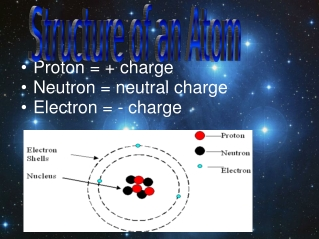DownloadDownload PresentationProton = + charge Neutron = neutral charge Electron = - charge

# Proton = + charge Neutron = neutral charge Electron = - charge

Download Presentation## Proton = + charge Neutron = neutral charge Electron = - charge

- - - - - - - - - - - - - - - - - - - - - - - - - - - E N D - - - - - - - - - - - - - - - - - - - - - - - - - - -
##### Presentation Transcript

1. Proton = + charge Neutron = neutral charge Electron = - charge

2. Ions Ions are atoms that have lost or gained one or more electrons giving them a positive or negative charge!

3. If an ion gains an electron it has a negative charge. If an ion looses an election it has a positive charge. For example: Na (Sodium), has originally 11 electrons but when an electron is lost it becomes a postive ion. Na +

4. What causes an atom to become an ion? • When an atom loses or gains one or more electrons it acquires a net electrical charge called an ion. • The net charge of an ion is found by subtracting the number of electrons from the number of protons • Charge of Ion = number of protons – number of electrons

5. A neutral magnesium atom (atomic number=12) has 12 protons/electrons. If it loses 2 electrons it becomes an ion with a charge of 2+. Number of protons 12 Number of electrons - 10 Charge of Ion 2+

6. Ion Electrons Protons 19. Cu²+ 20. F- 29 9 27 10

7. Isotopes • Isotopes are atoms that have the same number of protons but different numbers of neutrons • Most elements in the first two rows of the periodic table have at least 2 isotopes with one being more common than the other • In nature, elements are almost always found as a mixture of isotopes Hmm

8. Hydrogen Deuterium Tritium Isotopes of Hydrogen • For example, the most common isotope of hydrogen has no neutrons at all • There's also a hydrogen isotope called deuterium, • with one neutron, and another, tritium, with two neutrons. Most common isotope Neutron Proton

9. To identify an isotope more specifically, chemists add a number after the elements name. • ex. Carbon-11 Carbon-12 Carbon-14 • This number is called the isotope’s mass number and is the sum of the isotope’s number of protons and neutrons. • For example, an atom with 17 protons and 20 neutrons has a mass number of 37.

10. Q. What is the mass of the Nitrogen isotope with 7 protons and 12 neutrons? A. Nitrogen-19

11. Examples AtomProtonsNeutronsElectrons iodine-128 potassium-40 53 19 75 21 53 19

12. To name an isotope using chemical symbols • simply place the atom’s mass number to the upper left of the element symbol. For example 37Cl. #neutrons = atomic mass – atomic number 37 – 17 = 20 neutrons

13. How many protons, neutrons, and electrons are present? 56 26 STEP 1: The atomic number in the lower left corner = 26 protons. The difference between the mass number and the atomic number is 56 – 26 = 30. There are 30 neutrons. STEP 2: The charge on the ion, 2+ shows us that there are 2 more protons than electrons, indicating that there are 24 electrons. Fe 2+

14. Isotope Symbols Mass number (M) Ba2+ 141 Charge 56 Atomic number (Z)

15. Number of protons = Z Number of neutrons = M – Z charge = Z– Number of electrons